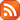Subscribe by RSS

# Topology Seminar

Thu., Apr. 14, 2022 1:00 p.m.

Location: RIC 208 and Live Stream

Speaker: Sacha Ikonicoff, University of Calgary

Zoom: https://uregina-ca.zoom.us/j/99127226830?pwd=bnFQR1R3UUdyWUxqSS9JMExMRlZwZz09

Abstract:

Divided power algebras were defined by H. Cartan in 1954 to study the homology of Eilenberg-MacLane spaces. They are commutative algebras endowed, for each integer n, with an additional monomial operation. Over a field of characteristic 0, this operation corresponds to taking each element to its n-th power divided by factorial of n. This definition does not make sense if the base field is of prime characteristic, yet Cartan's definition of divided power algebra applies in this situation as well. The notion of divided power algebra over a field of prime characteristic allows us to describe algebraic structures that appear in homology and homotopical​ algebra and has found applications in a wide array of mathematical domains, for instance in crystalline cohomology, and deformation theory.

In this talk, we will introduce the generalised definition of a divided power algebra over an operad given by B. Fresse in 2000. We will give a complete characterisation for generalised divided power algebras in terms of monomial operations and relations. We will show how to improve this characterisation to particular cases, including the case of a product of operads with distributive laws.